This post stem from an interesting discussion in the course W203: Exploring and Analyzing Data happened on July 16, 2015. The premise is a simple enough statistical puzzle at a glance - yet mainstream parametric hypothesis test procedures prove inadequate.

## Problem

Do Facebook users have higher median number of children than non-Facebook users?

(Data: 5000 sample-size survey)

As noted in the class, the research question here asks about population meidan as opposed the usual mean in typical statistical textbooks, normal techniques like T test and ANOVA are out of the window. We talked about possibly using either Wilcoxon rank sum test, Mood’s Median test or bootstrapping.

In this post, we ask a slightly different (easier) version of the question:

And we try to evaluate different tests in answering this question through simulation.

## Notations

Denote the r.v. of number of children of individuals who are Facebook users, and the number of children of individuals who are not Facebook users. Assume we have data from 2500 and 2500 , namely 2500 observations from each group.

vs.

## Notes on Median

For a real-valued random variable with CDF , the median is defined as the the real number such that:

or:

For with an absolutely continuous probability distribution, we have:

In general, median is not necessarily unique. For example, Bernoulli(0.5) does not have a single unique median, any satisfies the above defining properties, and therefore is a valid median. Also, at least one median always exist for any random variable, and the largest median is and smallest median is . See . In addition, the set of median is always a closed and bounded interval in in case it is not unique.

## When Student T Test is Suitable

T test assumes normal distribution with the same variance from both groups of observations. The Normal distribution’s median is the same as its mean. Therefore, if we are reasonably certain, the two populations are normal, we can use T test to test the equality of population median.

For out specific problem outlined previously, however, we cannot assume normal distribution - even before any normality test invalidates the assumption. The number of children for a given individual is discreet, non-negative, bounded and most likely not symmetrical. Hence, we suspect T test will perform poorly in answering the research question.

## Special Case 1: Binomial Population Distribution

We start our exploration simple: by assuming the population is follows a Binomial distribution, more to the point, a special class of Binomial distribution where the median is unique (when is an integer). To model number of children as a Binomial distribution is questionable, however, Binomial r.v. does have the desired property of discreet, non-negative values, and we can easily tweak and to have different distribution yet identical medians.

### Population Characteristics

Here are the assumptions for population distribution:

and

We have:

and is satisfied. The two populations are purposefully made distinct enough, has a positive skewness and has a negative skewness by design, which further invalidates normality assumption which is required for T test to be effective.

### Simulation Setup

Now lets prepare for the simulation. In R a test is usually a function that returns some object typically with the attribute $p.value. We can take advantage of this in designing our simulation interface. sim.run is just a dumb function take takes a test function, do a random sampling from our and populations (by specifying sample function sample.fun, which would return a matrix/data.frame of dimension , the first column being ’s and second being ’s) and return test’s p.value. # single run sim.run <- function(test, sample.fun, sample_size = 2500) { data <- sample.fun(sample_size) x <- data[ ,1] y <- data[ ,2] test(x,y)$p.value

}


For our Binomial populations, here’s the implementation of sample.fun

sample.binom <- function(n) {

cbind(

rbinom(n, 100, 0.15),

rbinom(n, 20, 0.75)

)

}


The Pmf for and are plotted below:

pmf.X <- data.frame(k = 0:100, prob = dbinom(0:100, size = 100, p = 0.15), variable = 'X')

pmf.Y <- data.frame(k = 0:20, prob = dbinom(0:20, size = 20, p = 15/20), variable = 'Y')

dat <- rbind(pmf.X, pmf.Y)

p <- ggplot(dat, aes(x = k, y = prob))

p + geom_segment(aes(xend = k, yend = 0), size=0.5) + ylab('p(k)') + facet_grid(. ~ variable)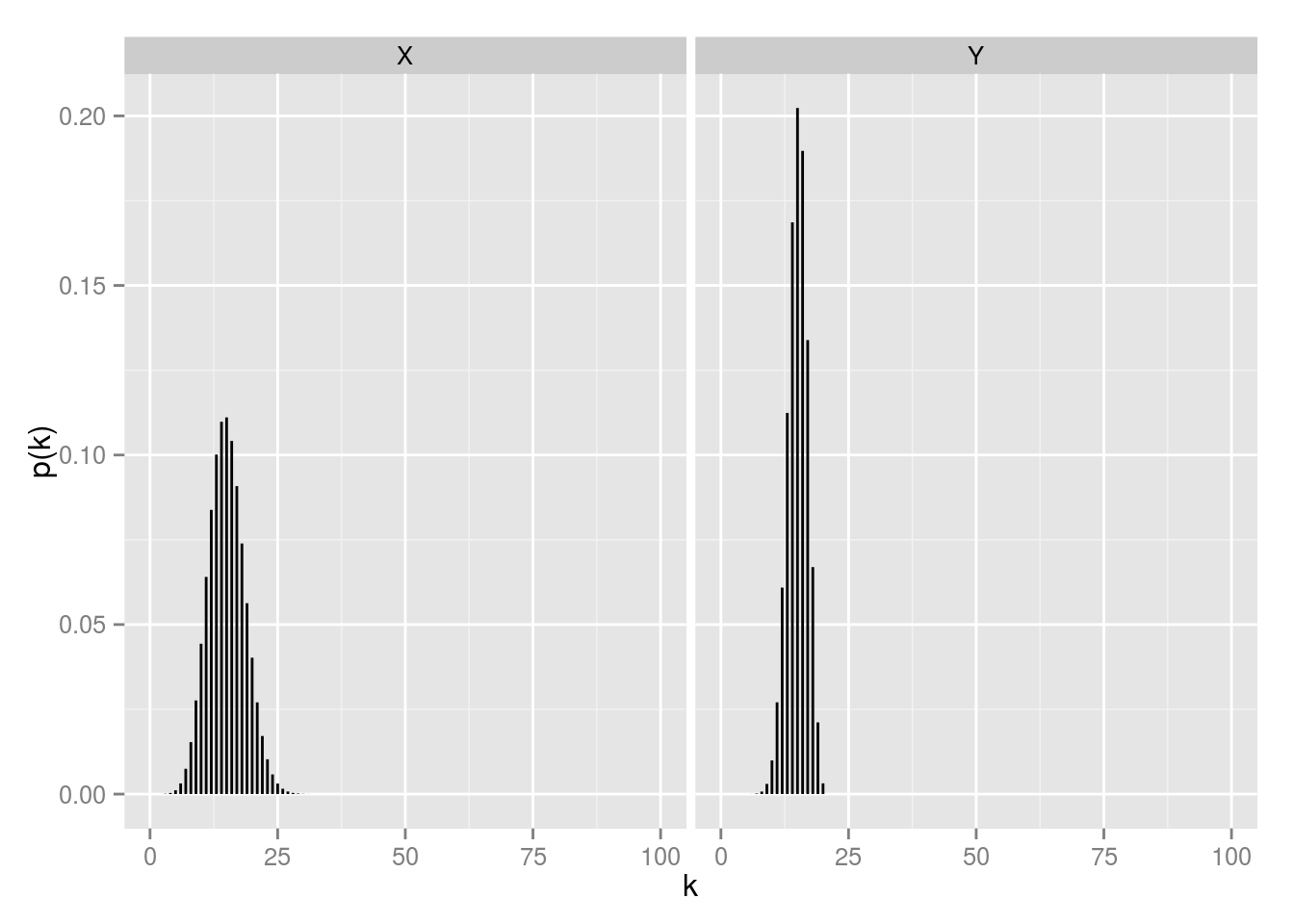### Implementing Tests

For T Test and Wilcox rank sum tests, we have readily available test functions in base R: t.test and wilcox.test. For Mood’s Test and Bootstrapped T Test, we need to implement them ourselves.

#### Mood’s Test

Let’s quickly go over what Mood’s Test is as it is lesser known (even its Wikipedia page is a stub!). This is a good intro. To recap:

1. Determine the overall median.

2. For each sample, count how many observations are greater than the overall median, and how many are equal to or less than it.

3. Put the counts from step 2 into a 2xk contingency table

sample1 sample2
greater than overall median
less than or equal to overall median
1. Perform a chi-square test on this table, testing the hypothesis that the probability of an observation being greater than the overall median is the same for all populations.

And here’s the code:

mood.test <- function(x, y) {

overall_mid <- median( c(x, y) )

nx <- length(x)

ny <- length(y)

nxbelow <- sum(x <= overall_mid)

nxabove <- sum(x > overall_mid)

nybelow <- sum(y <= overall_mid)

nyabove <- sum(y > overall_mid)

chisq.test( rbind( c(nxabove, nxbelow), c(nyabove, nybelow) ) )

}


#### Bootstrapped T Test

Given a sufficiently large sample size (in our case 2500 for and ), we can generate new samples by random sampling with replacement, this is the central idea of bootstrapping. For each set of the re-sampled data , we can compute a sample median . The sample median is an unbiased estimator of population median, therefore, the mean of all ’s follows a normal distribution asymptotically with the mean of population median, due to CLT - and this is a statistic we can perform T Test on.

In addition to Bootstrapping, we also consider subsampling. The key difference is when resampling, subsampling samples a smaller sample size and without replacement. The advantage of subsampling is that it is valid under much weaker conditions compared to the bootstrap.

The code:

medians.bootstrap <- function(x, k=1e3) {

n <- length(x)

replicate(k, expr=median(sample(x, n, replace=TRUE)))

}

medians.subsample <- function(x, k=1e3) {

n <- 100

replicate(k, expr=median(sample(x, n, replace=FALSE)))

}

t.test.bootstrap <- function(x, y) {

tryCatch({

t.test(medians.bootstrap(x), medians.bootstrap(y))

}, error=function(err) {

list(

p.value=NA

)

})

}

t.test.subsample <- function(x, y) {

tryCatch({

t.test(medians.subsample(x), medians.subsample(y))

}, error=function(err) {

list(

p.value=NA

)

})

}


### Simulations

With all building blocks, ready, now we run all five (T, Wilcox, Mood, Bootstrapped T, Subsample T) tests on 10000 random samples, and record the p-values. What should the distribution of simulation p-values look like? Recall the relation between Type I Error probability, alpha and p-value:

For , where is the r.v. for p-value in performing repeated tests on different samples from the same population. That’s the distribution function of a uniform r.v.

At this step, a problem surfaced. Since there are so few possible values sample observations could occupy, resampling using Bootstrapping essential produces the same sample median all the time, halting the subsequent T Test. Error message in R:

Error in t.test.default(c(1, 1), c(1, 1)) : data are essentially constant

Therefore, we have to drop Bootstrapping in favor of Subsampling.

p.values <- cbind(

replicate(n=1e4, expr=sim.run(t.test, sample.binom)),

replicate(n=1e4, expr=sim.run(wilcox.test, sample.binom)),

replicate(n=1e4, expr=sim.run(mood.test, sample.binom)),

replicate(n=1e4, expr=sim.run(t.test.subsample, sample.binom))

)

p.values <- as.data.frame(p.values)

names(p.values) <- c('T', 'Wilcox', 'Mood', 'Subsample')


### Kernel Density Plots of p-values

par(mfrow=c(2,2))

for(i in 1:4) {

p.value <- p.values[!is.na(p.values[ ,i]), i]

plot(density(p.value, from=0 ,to = 1), main=names(p.values)[i])

}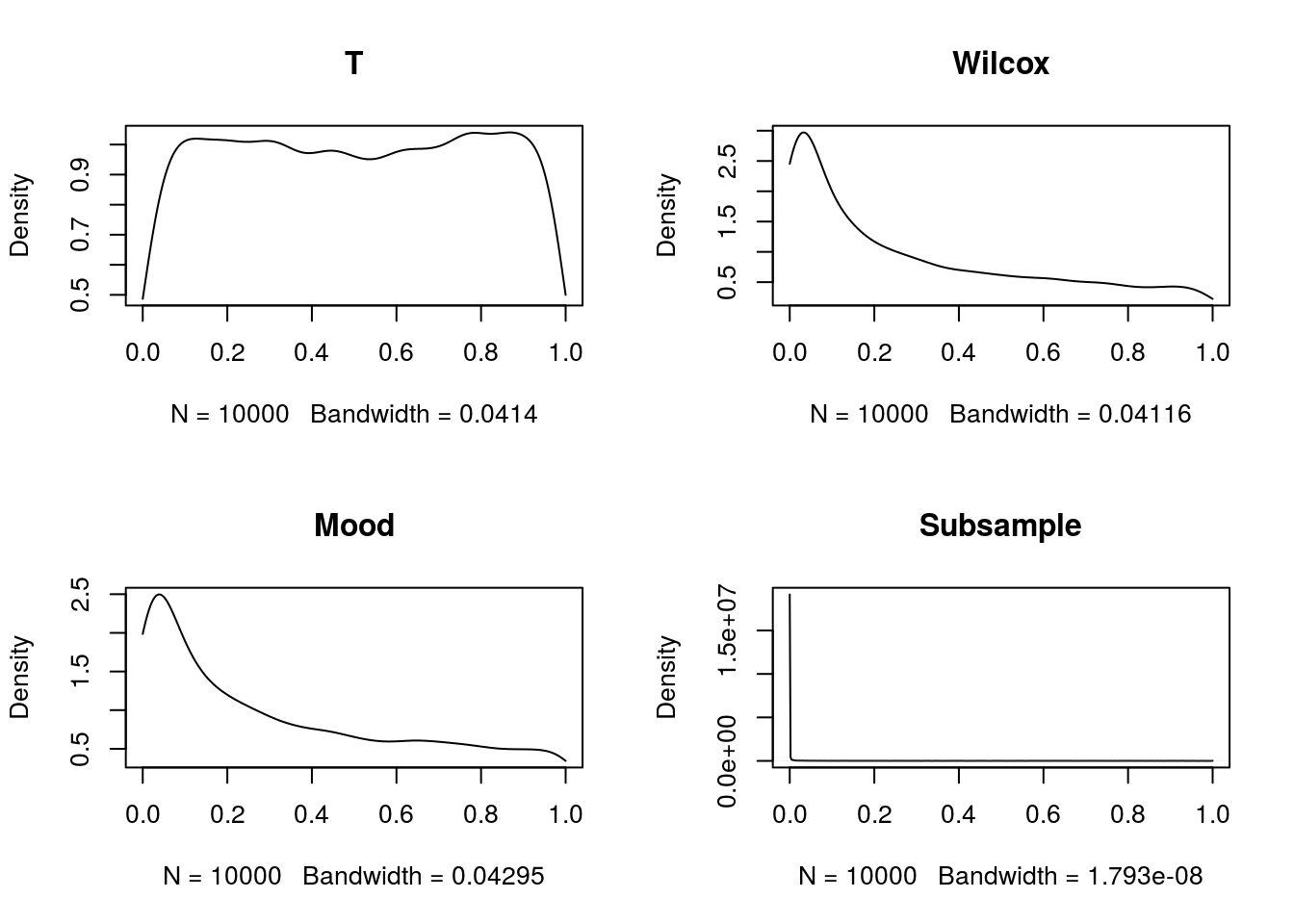### ECDF Plots of p-values

par(mfrow=c(2,2))

for(i in 1:4) {

p.value <- p.values[!is.na(p.values[ ,i]), i]

plot.ecdf(p.value, main=names(p.values)[i])

}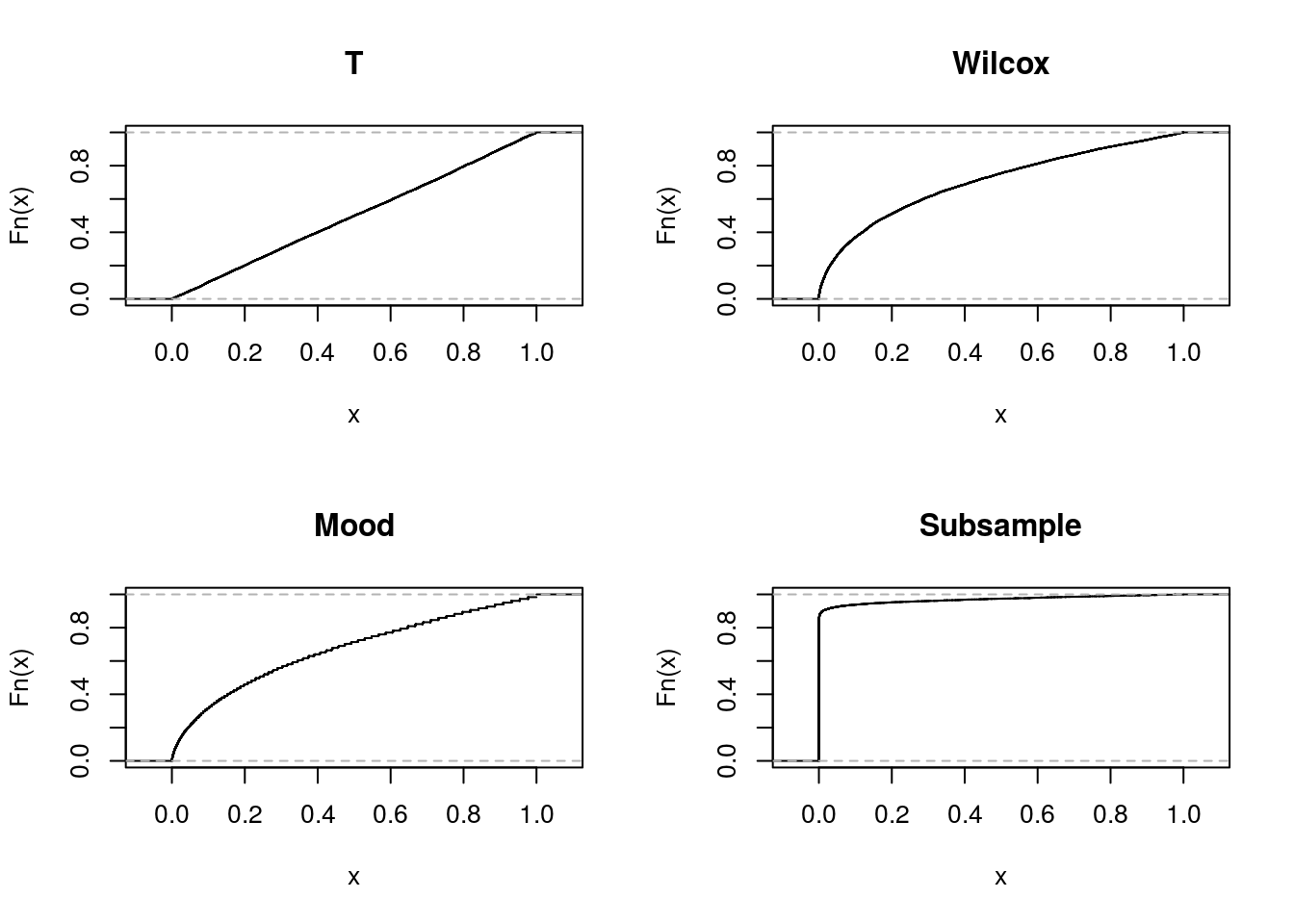### Conclusions for Binomial Population

For our specifically chosen population, T Test looks surprisingly good, its empirical p-values is the closest to uniform distribution among the four tests. The reason, of course is for Binomial distribution its mean and median is the same: , and T Test turns out to be very robust. A typical method to evaluate tests is Power function. In our context, rephrase the hypothesis as follows:

vs.

Power function is defined as:

when is true, i.e.

when is true, i.e.

where is the reject region of the test.

The ideal power function should have a value of 0 when and 1 when . For example, If our test is a coin flip, the corresponding power function is:

which is not very good.

The empirical power function values when is true () is, assuming a 5% :

• T Test: 0.0485

• Wilcox Test: 0.2614

• Mood Test: 0.2128

• Subsampling T Test: 0.9255

Clearly, T Test is the winner here, other tests have larger Type I error probabilities, and Subsampling T Tests is even worse than a coin flip test.

## Special Case 1: Binomial Population Distribution (Continued)

Now we consider the populations where is true:

sample.binom2 <- function(n, diff) {

cbind(

rbinom(n, 100, (15 + diff) / 100),

rbinom(n, 20, 0.75)

)

}


The goal of this section is to get a good picture of the shape of power functions, by controlling . We create a different sample function, withe additional parameter diff as the difference of population median. We shall consider .

power <- function(diff, runs=1e4) {

sample.fun <- function(n) {

sample.binom2(n, diff)

}

p.values <- cbind(

replicate(n=runs, expr=sim.run(t.test, sample.fun)),

replicate(n=runs, expr=sim.run(wilcox.test, sample.fun)),

replicate(n=runs, expr=sim.run(mood.test, sample.fun)),

replicate(n=runs, expr=sim.run(t.test.subsample, sample.fun))

)

p.values <- as.data.frame(p.values)

names(p.values) <- c('T', 'Wilcox', 'Mood', 'Subsample')

summarize(p.values,

T=mean(T < 0.05),

Wilcox=mean(Wilcox < 0.05),

Mood=mean(Mood < 0.05),

Subsample=mean(Subsample[!is.na(Subsample)] < 0.05))

}


Running the simulation for all values:

powers <- ldply(c(-5:-1, 1:5), power)

powers <- cbind(powers, c(-5:-1, 1:5))

names(powers) <- 'Median.Diff'

print(powers)

##    T Wilcox Mood Subsample Median.Diff
## 1  1      1    1         1          -5
## 2  1      1    1         1          -4
## 3  1      1    1         1          -3
## 4  1      1    1         1          -2
## 5  1      1    1         1          -1
## 6  1      1    1         1           1
## 7  1      1    1         1           2
## 8  1      1    1         1           3
## 9  1      1    1         1           4
## 10 1      1    1         1           5

All tests have very high powers.

## Special Case 2: Randomized Populations

### Population Characteristics

There are infinitely many ways of specifying population distribution of and . In this section, we restrict the potential values of both and to . The Pmf of can be specified by 10 params: where . Same goes for . Note the population median is not necessarily unique.

If there exists such that:

then the median is not unique, any number in is a population median. If we define median as , the sample median is an unbiased estimator of population median.

If no such exist, i.e. and then population median is . In this case, sample median is a biased estimator (but asymptotically unbiased).

Following the above definition of median (in case it’s not unique), in this section, we shall generate from an uniform distribution, then normalize them such that . Once the population of is chosen, we use the following procedure to choose ’s, such that :

1. If is not an integer (eg. ):
• for , choose from an uniform distribution, and normalize them such that:
• for , choose from an uniform distribution, and normalize them such that:
1. If is an integer
• choose and from Uniform(0, ) and Uniform(, 1)
• for , choose from Uniform distribution, and normalize so that they sum to
• for , choose from Uniform distribution, and normalize so that they sum to
• set to

Note in this process, scenario 1. will almost never happen. Also, since in general, sample median is biased, the subsampling method is probably not reliable.

### R Code for Random Populations

The following function generates the probabilities for

x.probs <- function() {

ps <- runif(11)

ps / sum(ps)

}


The following function pop.median and pop.mean computes the population median and mean given a vector of probabilities:

S <- lower.tri(diag(11), diag=TRUE)

pop.median <- function(probs) {

cdf <- as.vector(S %*% probs)

lower.half <- cdf[cdf < 0.5]

upper.half <- cdf[cdf > 0.5]

if(length(lower.half) + length(upper.half) < length(cdf)) {

m <- which(cdf == 0.5)

return(m - 0.5)

}

length(lower.half)

}

pop.mean <- function(probs) {

sum( probs*seq(0, 10) )

}


The following function takes care of scenario 1., and generates probabilities for given a non-integer median:

y.probs.1 <- function(m) {

m <- floor(m)

ps.lower <- runif(m)

ps.upper <- runif(11 - m)

ps.lower <- ps.lower / sum(ps.lower) / 2

ps.upper <- ps.upper / sum(ps.upper) / 2

c(ps.lower, ps.upper)

}


The following function takes care of scenario 2., and generates probabilities for given an integer median:

y.probs.2 <- function(m) {

pa <- runif(1, 0, 0.5)

pb <- runif(1, 0.5, 1)

ps.lower <- runif(m)

ps.upper <- runif(10 - m)

ps.lower <- ps.lower / sum(ps.lower) * pa

ps.upper <- ps.upper / sum(ps.upper) * (1 - pb)

c(ps.lower, pb-pa, ps.upper)

}


And together, they form the function to generate probabilities for :

y.probs <- function(m) {

if(m == floor(m)) {

return(y.probs.2(m))

} else {

return(y.probs.1(m))

}

}


Here’s how two randomly generated populations look like in their pmf:

pxs <- x.probs()

mx <- pop.median(pxs)

pys <- y.probs(mx)

pmf.X <- data.frame(k = 0:10, prob = pxs, variable = 'X')

pmf.Y <- data.frame(k = 0:10, prob = pys, variable = 'Y')

dat <- rbind(pmf.X, pmf.Y)

dat$k <- as.factor(dat$k)

p <- ggplot(dat, aes(x = k, y = prob))

p + geom_segment(aes(xend = k, yend = 0), size=4) + ylab('p(k)') + facet_grid(. ~ variable)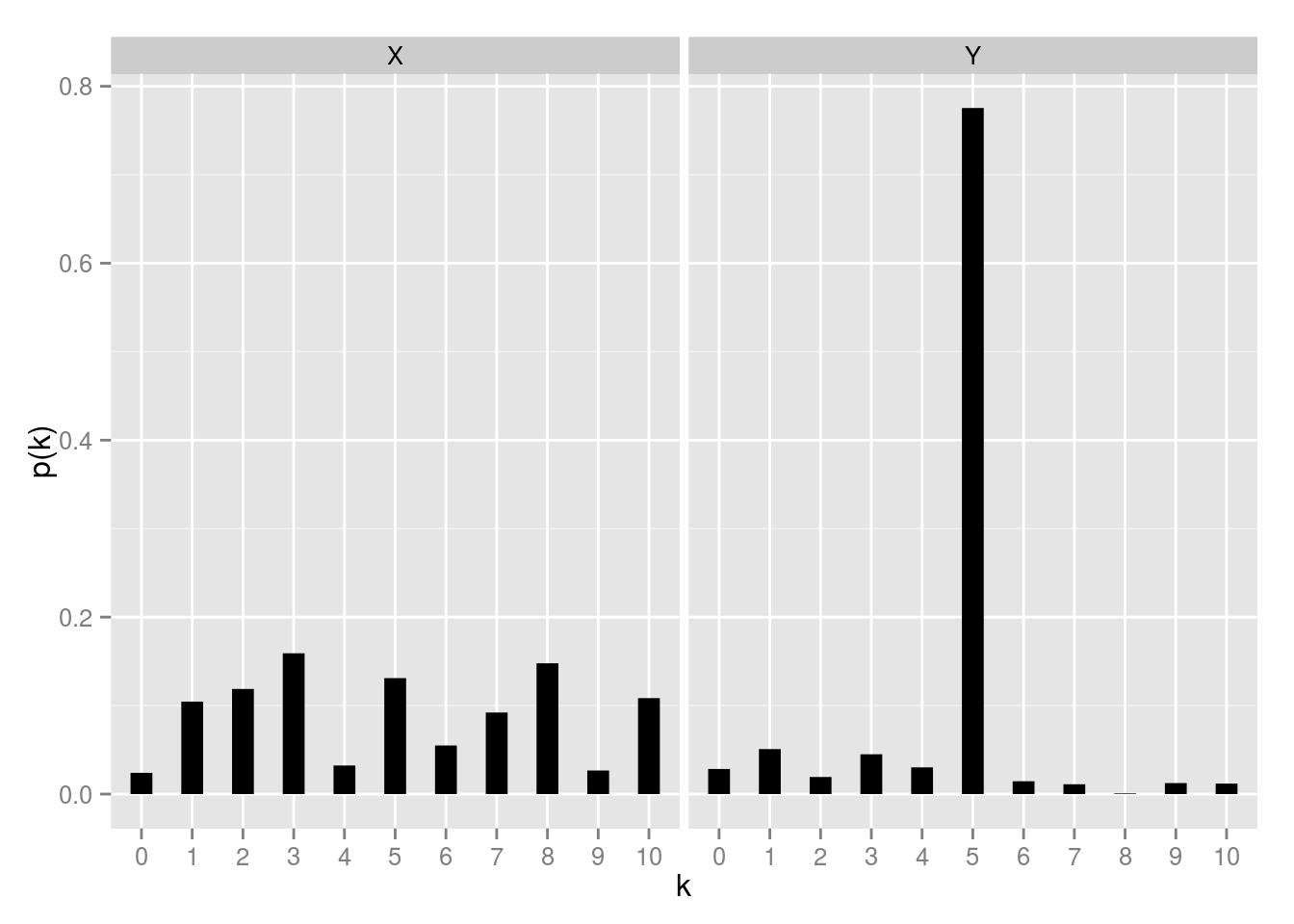print(pop.median(pxs))

##  5
print(pop.median(pys))

##  5

And we create a new sample function, which creates a pair of randomized populations with the same median, and sample from them:

sample.random_pop <- function(n) {

px <- x.probs()

m <- pop.median(px)

py <- y.probs(m)

cbind(

sample(x = seq(0, 10), n, replace = T, prob=px),

sample(x = seq(0, 10), n, replace = T, prob=py)

)

}


### Simulation

p.values <- cbind(

replicate(n=1e4, expr=sim.run(t.test, sample.random_pop)),

replicate(n=1e4, expr=sim.run(wilcox.test, sample.random_pop)),

replicate(n=1e4, expr=sim.run(mood.test, sample.random_pop)),

replicate(n=1e4, expr=sim.run(t.test.subsample, sample.random_pop))

)

p.values <- as.data.frame(p.values)

names(p.values) <- c('T', 'Wilcox', 'Mood', 'Subsample')


### Kernel Density Plots of p-values

par(mfrow=c(2,2))

for(i in 1:4) {

p.value <- p.values[!is.na(p.values[ ,i]), i]

plot(density(p.value, from=0 ,to = 1), main=names(p.values)[i])

}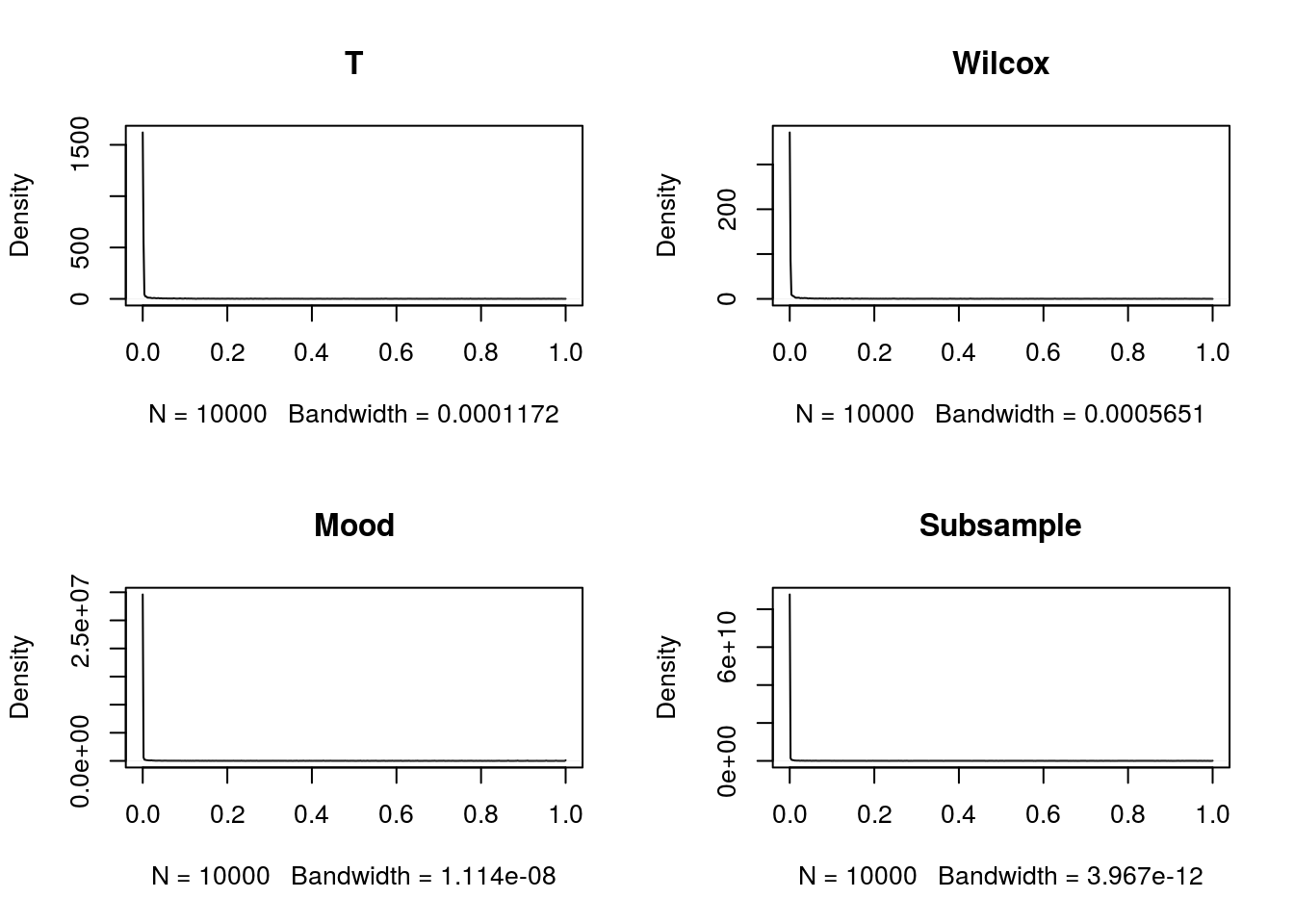### ECDF Plots of p-values

par(mfrow=c(2,2))

for(i in 1:4) {

p.value <- p.values[!is.na(p.values[ ,i]), i]

plot.ecdf(p.value, main=names(p.values)[i])

}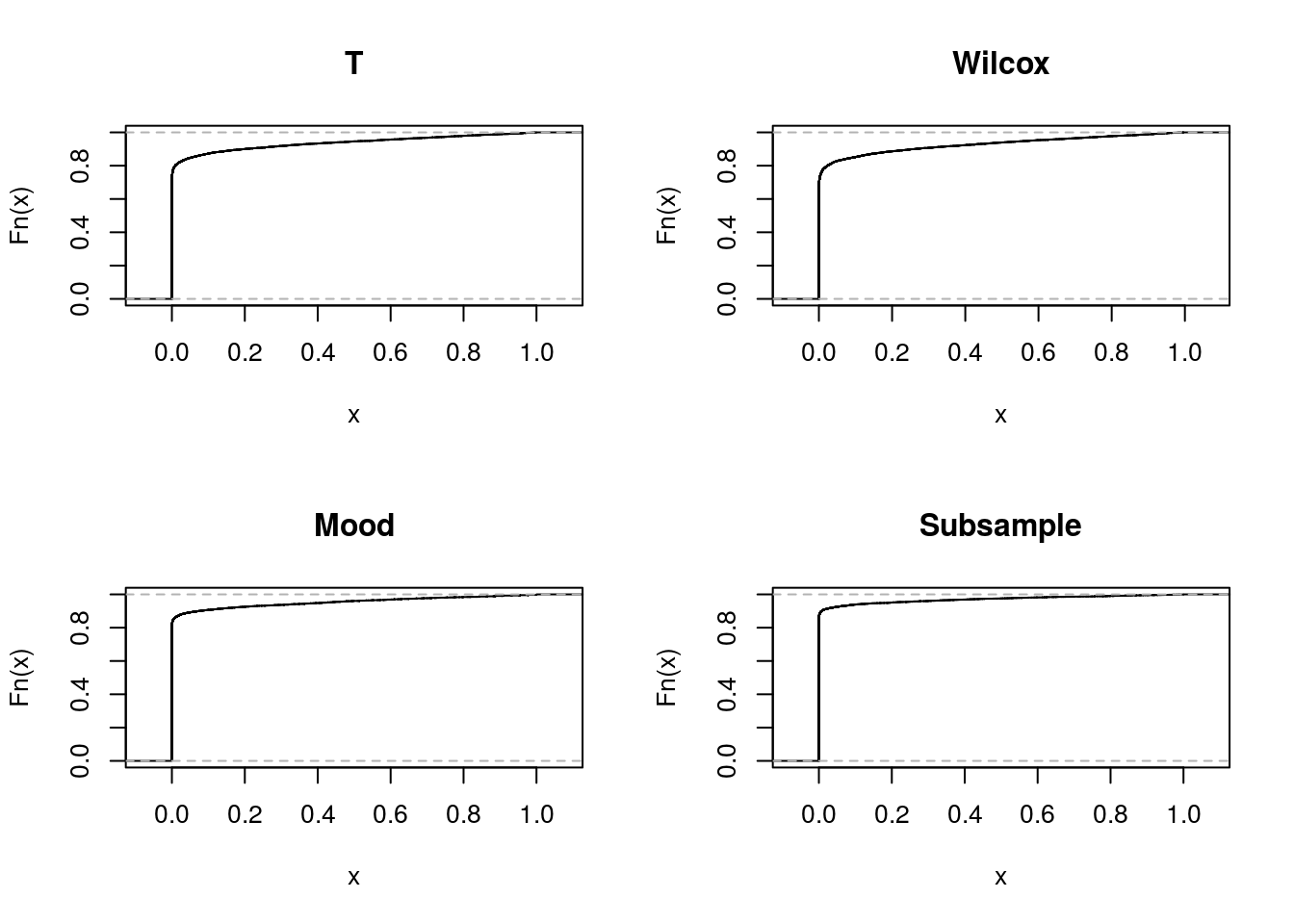### Conclusion

All of these look pretty bad.

### Trouble Shooting

Let’s generate two random populations:

set.seed(123)

pxs <- x.probs()

m <- pop.median(pxs)

pys <- y.probs(m)


Both populations have a median of 6, the mean for is 5.3046801 and 5.4742832 for . The mean and median is not the same for the population, therefore, we expect T Test to perform poorly. To get a sense of the population distribution, we plot their pmf:

pmf.X <- data.frame(k = 0:10, prob = pxs, variable = 'X')

pmf.Y <- data.frame(k = 0:10, prob = pys, variable = 'Y')

dat <- rbind(pmf.X, pmf.Y)

dat$k <- as.factor(dat$k)

p <- ggplot(dat, aes(x = k, y = prob))

p + geom_segment(aes(xend = k, yend = 0), size=4) + ylab('p(k)') + facet_grid(. ~ variable)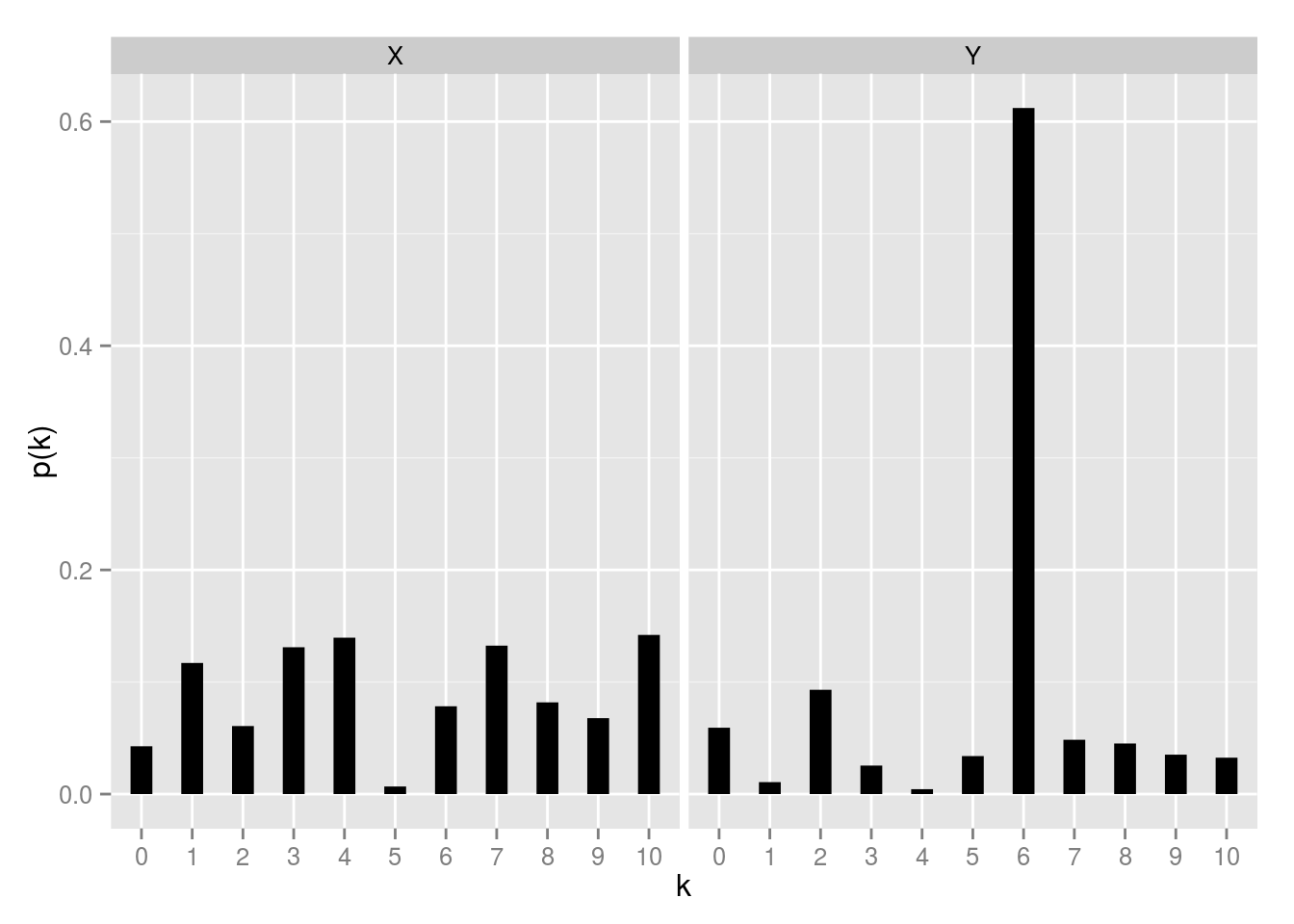and have very different distributions, even if they share the same median. Keep these two populations fixed, we repeated sample from them using the following sample function:

sample.random_pop2 <- function(n) {

cbind(

sample(x = seq(0, 10), n, replace = T, prob=pxs),

sample(x = seq(0, 10), n, replace = T, prob=pys)

)

}


And Run the simulation

p.values <- cbind(

replicate(n=1e4, expr=sim.run(t.test, sample.random_pop)),

replicate(n=1e4, expr=sim.run(wilcox.test, sample.random_pop)),

replicate(n=1e4, expr=sim.run(mood.test, sample.random_pop)),

replicate(n=1e4, expr=sim.run(t.test.subsample, sample.random_pop))

)

p.values <- as.data.frame(p.values)

names(p.values) <- c('T', 'Wilcox', 'Mood', 'Subsample')

par(mfrow=c(2,2))

for(i in 1:4) {

p.value <- p.values[!is.na(p.values[ ,i]), i]

plot(density(p.value, from=0 ,to = 1), main=names(p.values)[i])

}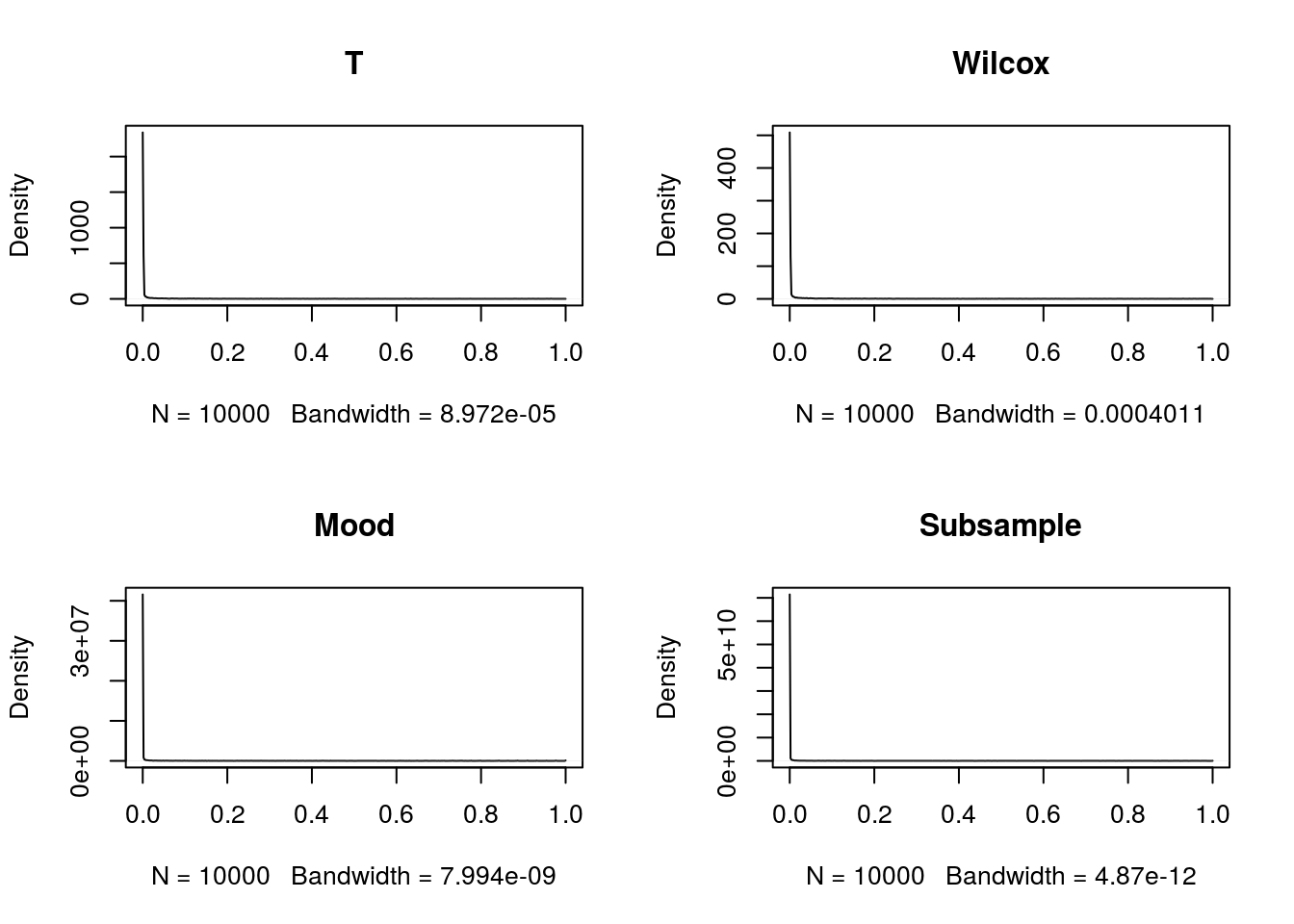#### Problem With Mood Test

One thing we notice is since many and observations will take the value of their population median 6. In the case of Mood Test, under (), the following is assumed to be true for the Chi-sq test to work:

This would work well for absolute continuously distributions, but it’s easy to verify this is not the case in our randomly generated populations, instead all we can say is:

and

The difference between (0.5333528) and (0.7795565) non-zero and quite large.

#### Problem With Subsampling

The first thing to keep notice is we choose a subsample size of 100 throughout this exercise, and we know the sample median could be biased, and this could cause problems. To illustrate the biasness of sample median, we shall draw samples from size ranging from 10 to 100000, and plot the sample median:

sizes <- floor(c(seq(50, 950, by=50), 10^(seq(3,5,by=0.2))))

medians <- sapply(sizes,

function(n) mean(replicate(1e3, expr=median(sample(x = seq(0, 10), n, replace = T, prob=pxs)))))

sample.medians <- data.frame(

size=sizes,

median=medians

)

p <- ggplot(sample.medians)

p <- p + geom_point(aes(x=size, y=median)) +

geom_line(aes(x=size, y=median)) +

geom_hline(yintercept=m) +

geom_text(aes(0,m,label = 'Population Median', hjust = -2, vjust=1))

plot(p)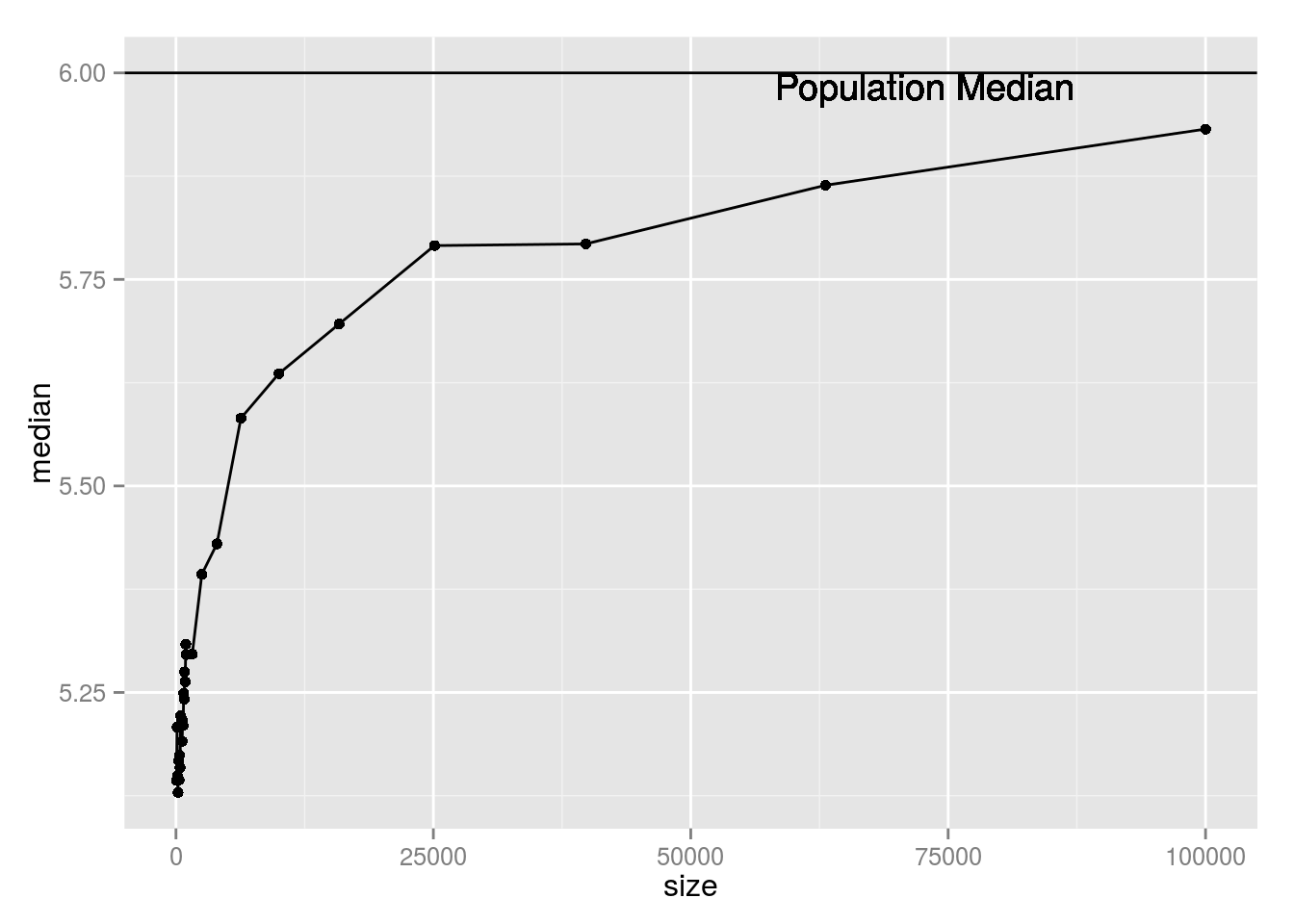For however, the bias is pretty-much non-existent, due to the fact (0.6121182) is very large.

medians <- sapply(sizes,

function(n) mean(replicate(1e3, expr=median(sample(x = seq(0, 10), n, replace = T, prob=pys)))))

sample.medians <- data.frame(

size=sizes,

median=medians

)

p <- ggplot(sample.medians)

p <- p + geom_point(aes(x=size, y=median)) +

geom_line(aes(x=size, y=median)) +

geom_hline(yintercept=m) +

geom_text(aes(0,m,label = 'Population Median', hjust = -2, vjust=1))

plot(p)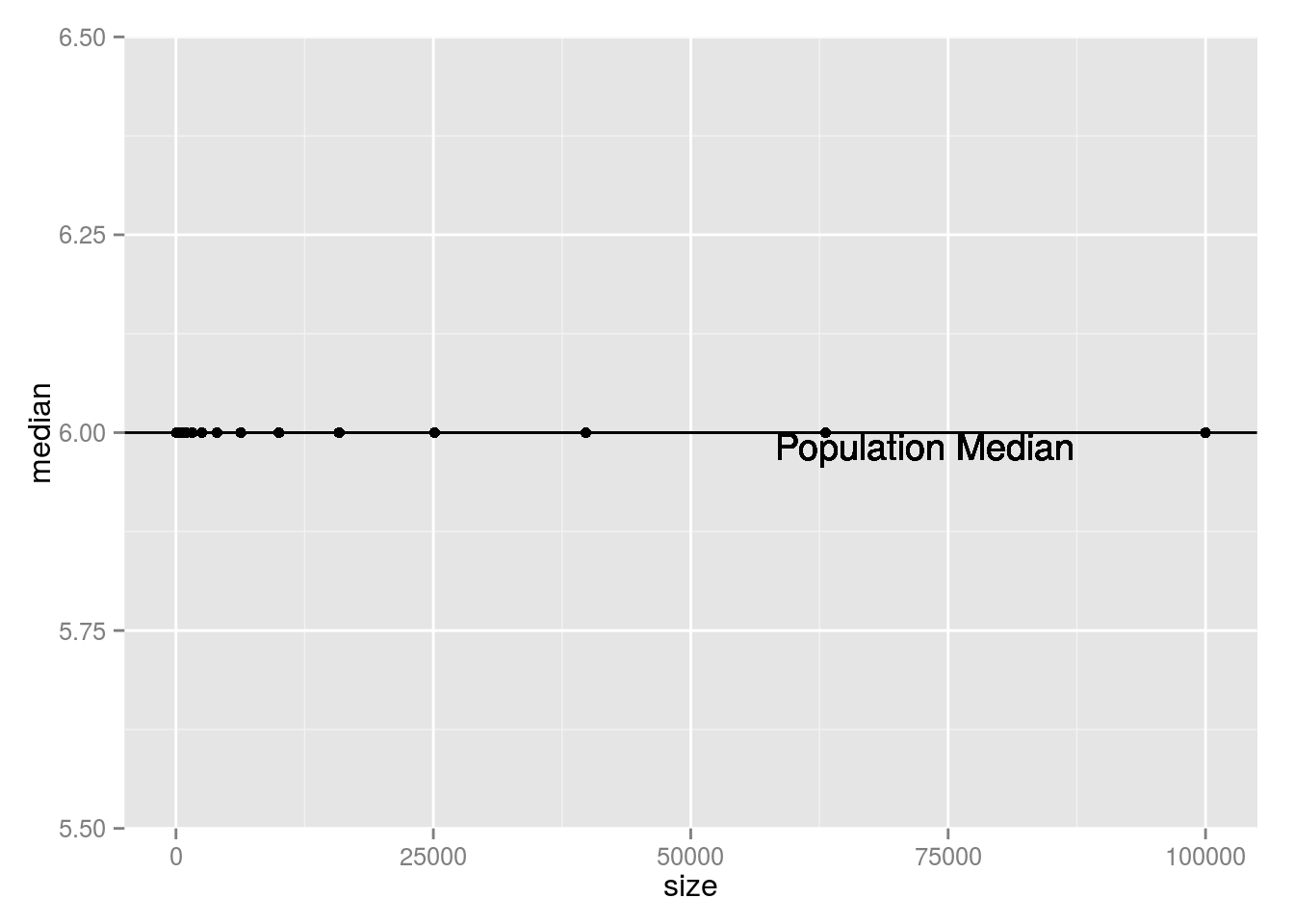Due to the bias of sample median in , the subsampling T test will reject for any reasonable subsample size we choose.

## Special Case 3: Random Population with Unbaised Sample Median Statistic

The failure in previous section hints for another line of experimentation: populations which have unbiased sample median statistics, in which case the subsampling method would’ve been much more potent. If we assume some population, with probabilities of ’s, whose sample median is an unbiased estimator of for all sample size ’s, this must hold true when . The sample median when is , which is also the sample mean.

where is population mean

Therefore, we must have: , population median and mean is the same. Turns out the unbiased sample median assumption is a pretty strong restriction. (Under which T Test would’ve also performed better)

It’s easy to prove if the distribution of is symmetrical, the above property is satisfied, some asymmetrical distributions also satisfies the same property, for example, Binomial distribution where is an integer.

### One Example

To satisfy the above restriction, one quick example we can think of is, follows a uniform discreet distribution in , and follows . Both have their mean and median equal to 5, yet their distribution is very different.

pxs <- rep(1/11, 11)

pys <- dbinom(c(0:10), 10, 0.5)


Their Pmfs are plotted below:

pmf.X <- data.frame(k = 0:10, prob = pxs, variable = 'X')

pmf.Y <- data.frame(k = 0:10, prob = pys, variable = 'Y')

dat <- rbind(pmf.X, pmf.Y)

dat$k <- as.factor(dat$k)

p <- ggplot(dat, aes(x = k, y = prob))

p + geom_segment(aes(xend = k, yend = 0), size=4) + ylab('p(k)') + facet_grid(. ~ variable)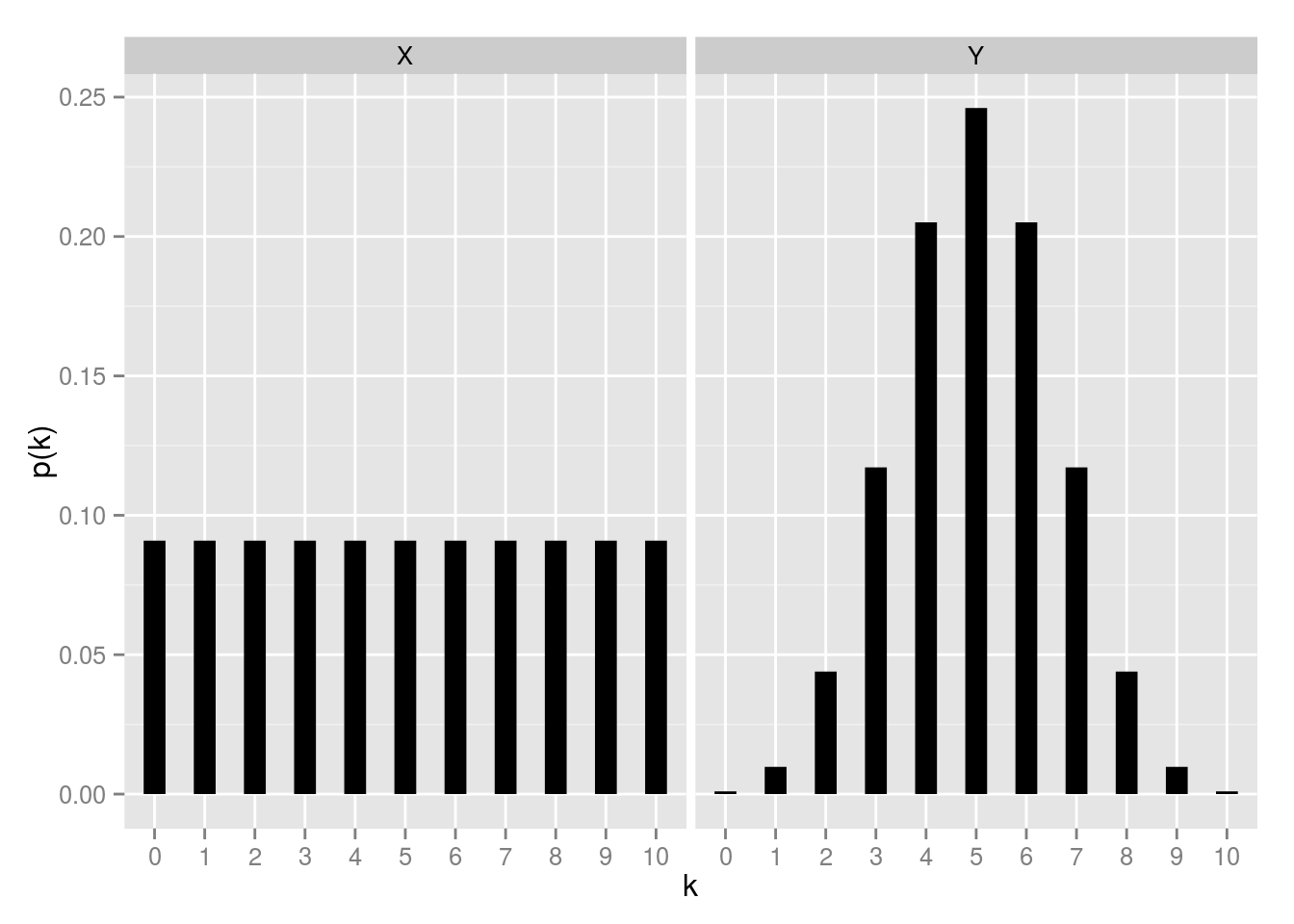### Simulation

sample.random_pop3 <- sample.random_pop2  # global variable pxs and pys have changed, so we can reuse this function

p.values <- cbind(

replicate(n=1e4, expr=sim.run(t.test, sample.random_pop3)),

replicate(n=1e4, expr=sim.run(wilcox.test, sample.random_pop3)),

replicate(n=1e4, expr=sim.run(mood.test, sample.random_pop3)),

replicate(n=1e4, expr=sim.run(t.test.subsample, sample.random_pop3))

)

p.values <- as.data.frame(p.values)

names(p.values) <- c('T', 'Wilcox', 'Mood', 'Subsample')


### Kernel Density Plots of p-values

par(mfrow=c(2,2))

for(i in 1:4) {

p.value <- p.values[!is.na(p.values[ ,i]), i]

plot(density(p.value, from=0 ,to = 1), main=names(p.values)[i])

}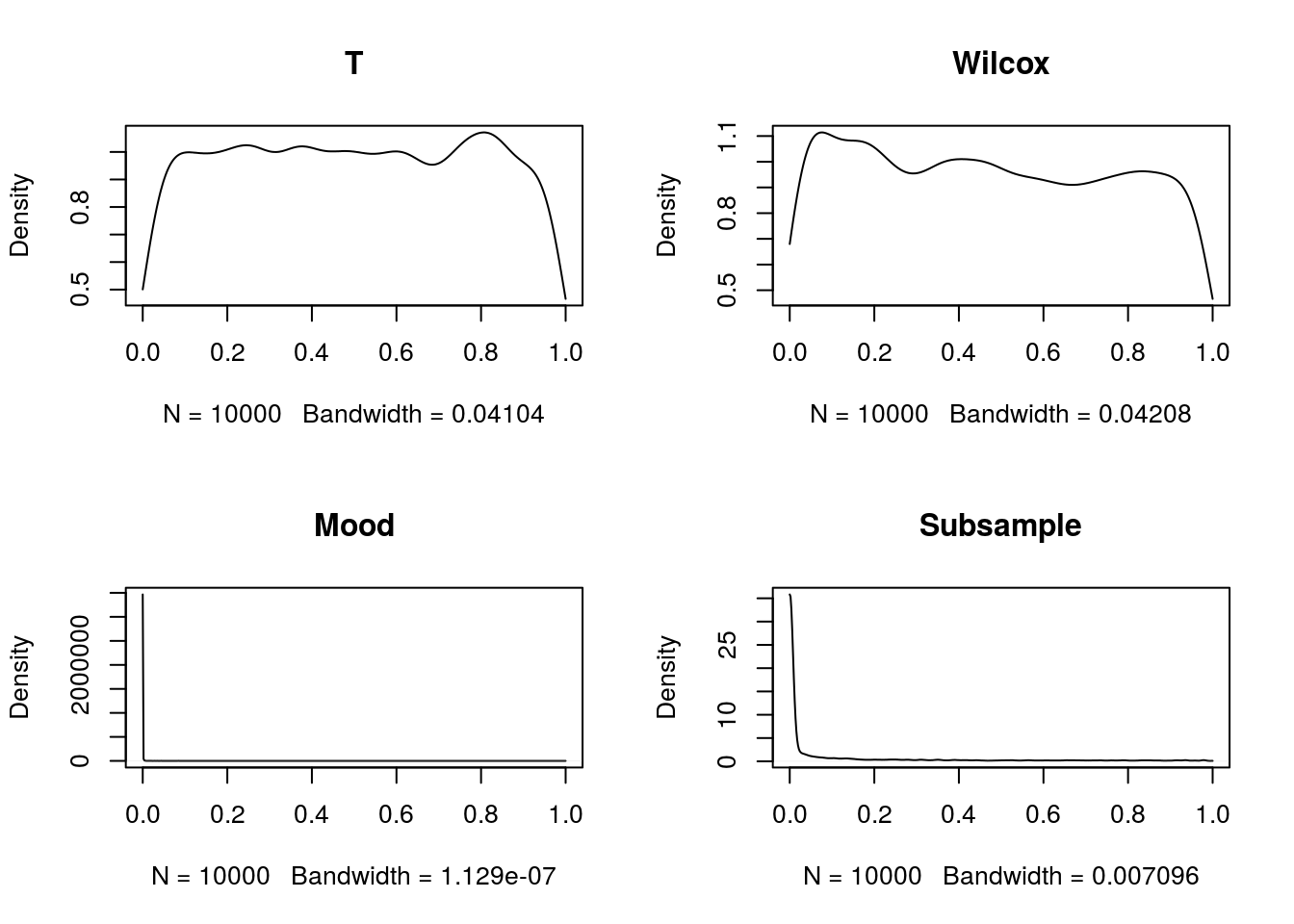### ECDF Plots of p-values

par(mfrow=c(2,2))

for(i in 1:4) {

p.value <- p.values[!is.na(p.values[ ,i]), i]

plot.ecdf(p.value, main=names(p.values)[i])

}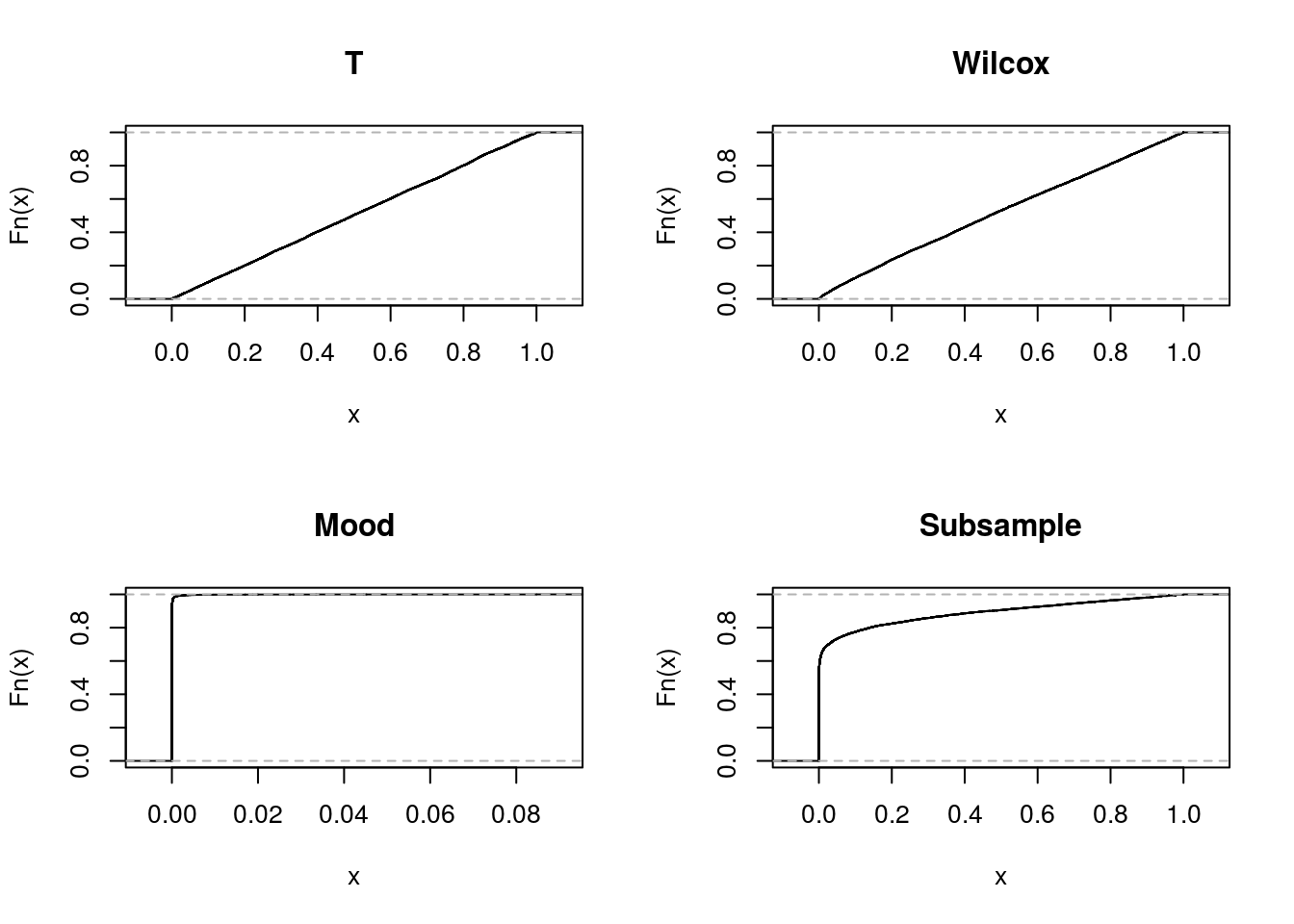### Type I Error Probabilities

The empirical power function values when is true is, assuming a 5% :

• T Test: 0.049

• Wilcox Test: 0.0703

• Mood Test: 0.9999

• Subsampling T Test: 0.7335

T Test and Wilcox rank sum both look good. For the same reason we have identified before Mood Test prove to be inadequate - surprising ly subsampling method does not do well at all, the reasons are yet to be analyzed.

## Summary

Throughout this analysis, we have tried various setup of different discreet populations, and run simulations on randomly sampled data from them. Here’s a few takeaways:

• If we know nothing about the population, all of the proposed tests are not reliable
• Mood Test tends to reject more often, it might be a good test for contentious distributions, but turns out to be a poor fit for discreet distributions, which can have identical medians yet fail to satisfy Mood’s Test condition
• Subsampling/Bootstrapping tend to over-reject as well, especially when sample median is biased
• When population mean and population median can be assumed to be equal, T Test is very robust despite its obvious theoretical shortcomings
• Wilcox rank sum test is a general close second following T Test, and has good robustness

## Footnotes

 MIDS program: http://datascience.berkeley.edu/